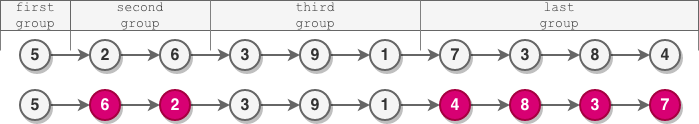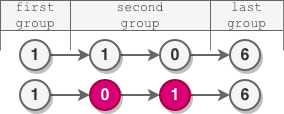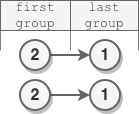• Contents

# For Solution

You are given the `head` of a linked list.

The nodes in the linked list are sequentially assigned to non-empty groups whose lengths form the sequence of the natural numbers (`1, 2, 3, 4, ...`). The length of a group is the number of nodes assigned to it. In other words,

• The `1st` node is assigned to the first group.
• The `2nd` and the `3rd` nodes are assigned to the second group.
• The `4th``5th`, and `6th` nodes are assigned to the third group, and so on.

Note that the length of the last group may be less than or equal to `1 + the length of the second to last group`.

Reverse the nodes in each group with an even length, and return the `head` of the modified linked list.

Example 1:```Input: head = [5,2,6,3,9,1,7,3,8,4]
Output: [5,6,2,3,9,1,4,8,3,7]
Explanation:
- The length of the first group is 1, which is odd, hence no reversal occurrs.
- The length of the second group is 2, which is even, hence the nodes are reversed.
- The length of the third group is 3, which is odd, hence no reversal occurrs.
- The length of the last group is 4, which is even, hence the nodes are reversed.
```

Example 2:```Input: head = [1,1,0,6]
Output: [1,0,1,6]
Explanation:
- The length of the first group is 1. No reversal occurrs.
- The length of the second group is 2. The nodes are reversed.
- The length of the last group is 1. No reversal occurrs.
```

Example 3:```Input: head = [2,1]
Output: [2,1]
Explanation:
- The length of the first group is 1. No reversal occurrs.
- The length of the last group is 1. No reversal occurrs.
```

Example 4:

```Input: head = 
Output: 
Explanation: There is only one group whose length is 1. No reversal occurrs.
```

Constraints:

• The number of nodes in the list is in the range `[1, 105]`.
• `0 <= Node.val <= 105`